### 15年5700亿，双色球16227注一等奖数据中，我们发现了这些秘密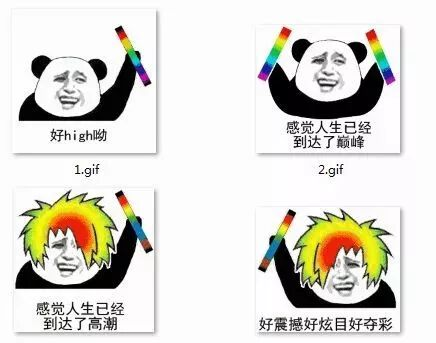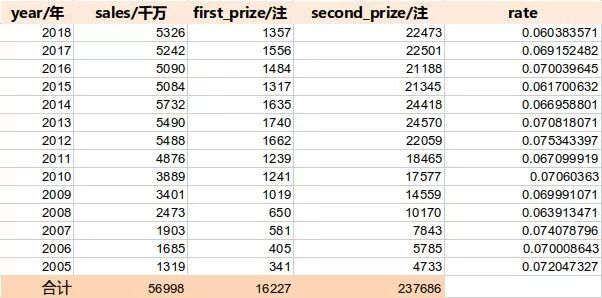▲2005-2018年，双色球的数据统计

▲视频来源：飞碟说

01 直方图解读历年中奖注数

1. matplotlib.pyplot.bar(left, height, alpha=1, width=0.8, color=, edgecolor=, label=, lw=3)

• left：x轴的位置序列，一般采用arange函数产生一个序列；

• height：y轴的数值序列，也就是柱形图的高度，一般就是我们需要展示的数据；

• alpha：透明度

• width：为柱形图的宽度，一般这是为0.8即可；

• color或facecolor：柱形图填充的颜色；

• edgecolor：图形边缘颜色

• label：解释每个图像代表的含义

• linewidth or linewidths or lw：边缘or线的宽度

2. matplotlib.pyplot.legend()

plt.legend()函数主要的作用就是给图加上图例，plt.legend([x,y,z])里面的参数使用的是list的的形式，默认情况下是将label值赋给它。

``import numpy as npimport matplotlib.pyplot as pltimport pandas as pddef DrawHistogram(read_name):    #读取数据    read_name = r"C:\Users\Administrator\Desktop\ssq.csv"    fp = pd.read_csv(read_name)    first_prize = fp.first_prize    second_prize = fp.second_prize    #配置图形参数    ind = np.arange(len(first_prize))    width = 0.5    fig, ax = plt.subplots()    rects1 = ax.bar(ind - width/2, first_prize, width, color='SkyBlue', label='First')    rects2 = ax.bar(ind + width/2, second_prize, width,color='IndianRed', label='Second')    ax.set_ylabel('Stakes')    ax.set_title('Stakes by year and rank')    plt.xticks(ind,(18,17,16,15,14,13,12,11,10,9,8,7,6,5))    ax.legend()     plt.show()if __name__=='__main__':   DrawHistogram(r"C:\Users\Administrator\Desktop\ssq.csv")``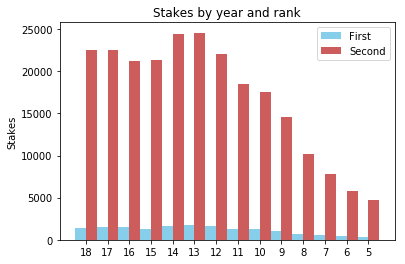▲历年一等奖、二等奖中奖数

02 气泡图解读销售额的大小，与一等奖的占比率并没有关系

• scatter(x,y) 在向量 x 和 y 指定的位置创建一个包含圆形的散点图。该类型的图形也称为气泡图。

• scatter(x,y,sz) 指定圆大小。要绘制大小相等的圆圈，请将 sz 指定为标量。要绘制大小不等的圆，请将 sz 指定为长度等于 x 和 y 的长度的向量。

• scatter(x,y,sz,c) 指定圆颜色。要以相同的颜色绘制所有圆圈，请将 c 指定为颜色名称或 RGB 三元数。要使用不同的颜色，请将 c 指定为向量或由 RGB 三元数组成的三列矩阵。

``import pandas as pdimport numpy as npimport seaborn as snsimport matplotlib.pyplot as pltdef DrawBubble(read_name):    #读取数据    fp = pd.read_csv(read_name)    x = fp.sales    y = fp.rate    z = fp.rate    #配置参数    sns.set(style = "whitegrid")    cm = plt.cm.get_cmap('RdYlBu')    fig,ax = plt.subplots(figsize = (12,10))    bubble = ax.scatter(x, y , s = (z - np.min(z) + 0.1) * 3000, c = z, cmap = cm, linewidth = 0.5, alpha = 0.5)    ax.grid()    fig.colorbar(bubble)     ax.set_xlabel('sales of year', fontsize = 15)    ax.set_ylabel('rate of something', fontsize = 15)    plt.show()if __name__=='__main__':    DrawBubble(r"C:\Users\Administrator\Desktop\ssq.csv")``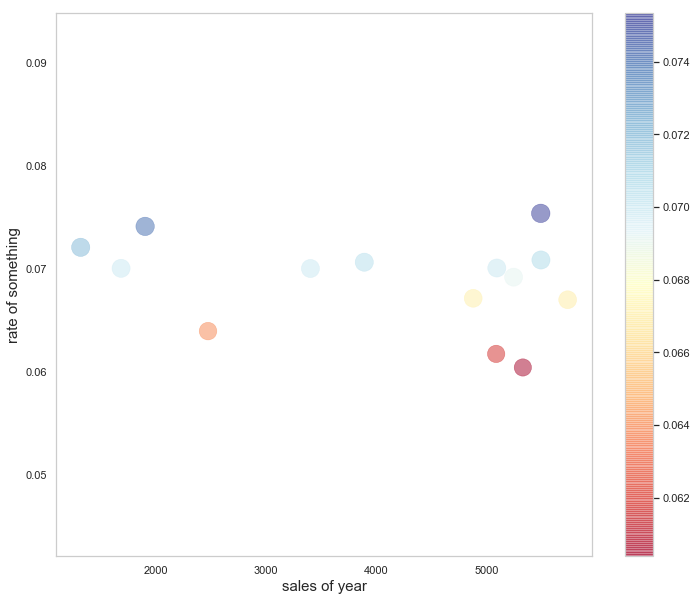▲历年销售额与一等奖的中奖占比率之间关系

03 用Python画一张好看的气泡图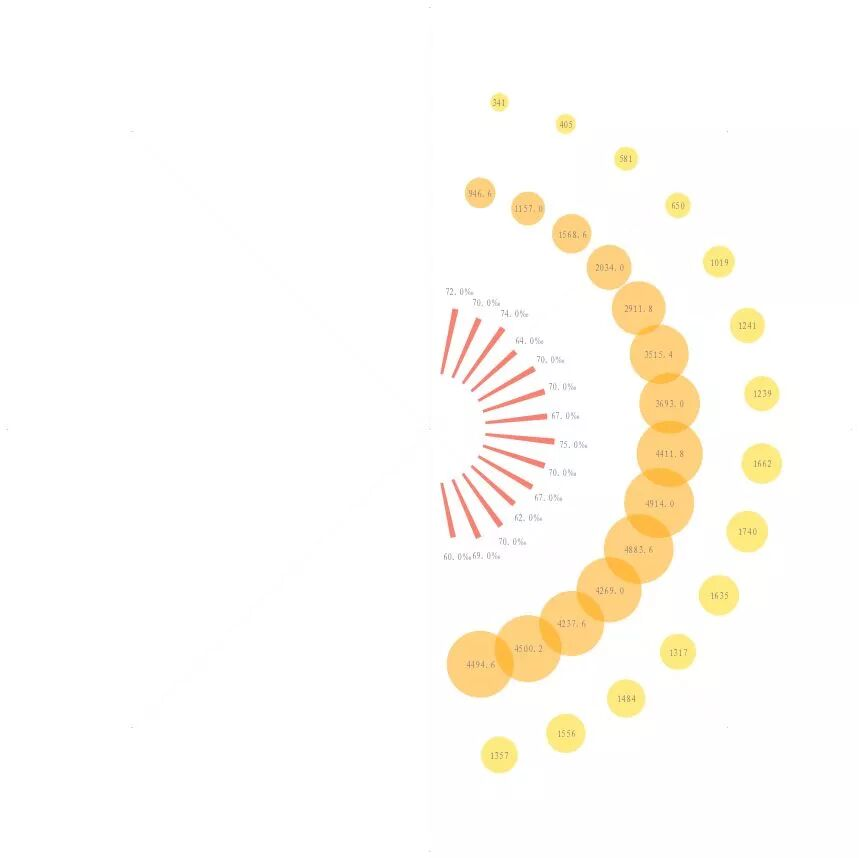``import numpy as npimport pandas as pdimport matplotlib.pyplot as pltimport matplotlib.font_manager#读取数据read_name = r"C:\Users\Administrator\Desktop\ssq.csv"fp = pd.read_csv(read_name)first_prize = fp.first_prizesecond_prize = fp.second_prizerate = fp.rate#一等奖中奖注数S1=first_prizex1=[(3/2)*np.pi+np.pi/(len(S1)+1)*(i+1) for i in range(2*(len(S1)+1)) if i<len(S1)]y1=[180 for i in range(2*(len(S1)+1)) if i<len(S1) ]#为了画出效果比例好看，将二等奖注数缩小5倍来表示S2=second_prize/5x2=[(3/2)*np.pi+np.pi/(len(S1)+1)*(i+1) for i in range(2*(len(S1)+1)) if i<len(S1)]y2=[130 for i in range(2*(len(S1)+1)) if i<len(S1) ]H1=[60/2,69/2,70/2,62/2,67/2,70/2,75/2,67/2,70/2,70/2,64/2,74/2,70/2,72/2]W1=[0.05 for i in range(2*(len(S1)+1)) if i<len(S1)]B=[30 for i in range(2*(len(S1)+1)) if i<len(S1)]fig=plt.figure(figsize=(13.44*2,7.5*2),facecolor='w')#建立一个坐标系，projection='polar'表示极坐标ax = fig.add_subplot(111,projection='polar',facecolor='w')ax.scatter(x=x1,y=y1,s=S1,color='Gold',alpha=0.5,linewidths=0)ax.scatter(x=x2,y=y2,s=S2,color='Orange',alpha=0.5,linewidths=0)ax.bar(x=x1, height=H1, width=W1,bottom=B,color='Salmon')plt.ylim(0,220)#去掉坐标轴的为外面一层粗的线ax.spines['polar'].set_visible(False)plt.grid(color='gray', linestyle=':', linewidth=1,which='major',axis='x',alpha=0.1)ax.set_yticks([])ax.tick_params(axis='x',labelsize=0)font=matplotlib.font_manager.FontProperties(fname=r'E:\project\wordcloud\simfang.ttf')for i in range(len(x1)):    ax.text(x=x1[i],y=y1[i],s=S1[i],color='gray',ha='center', va= 'center',fontsize=12,fontproperties=font)for i in range(len(x1)):    ax.text(x=x1[i],y=y2[i],s=S2[i],color='gray',ha='center', va= 'center',fontsize=12,fontproperties=font)for i in range(len(x1)):    ax.text(x=x1[i],y=H1[i]+40,s="{}‰".format(H1[i]*2) ,color='gray',ha='center', va= 'center',fontsize=12,fontproperties=font)plt.show()fig.savefig('D:\\test.png',dpi=400,bbox_inches='tight',transparent=False)``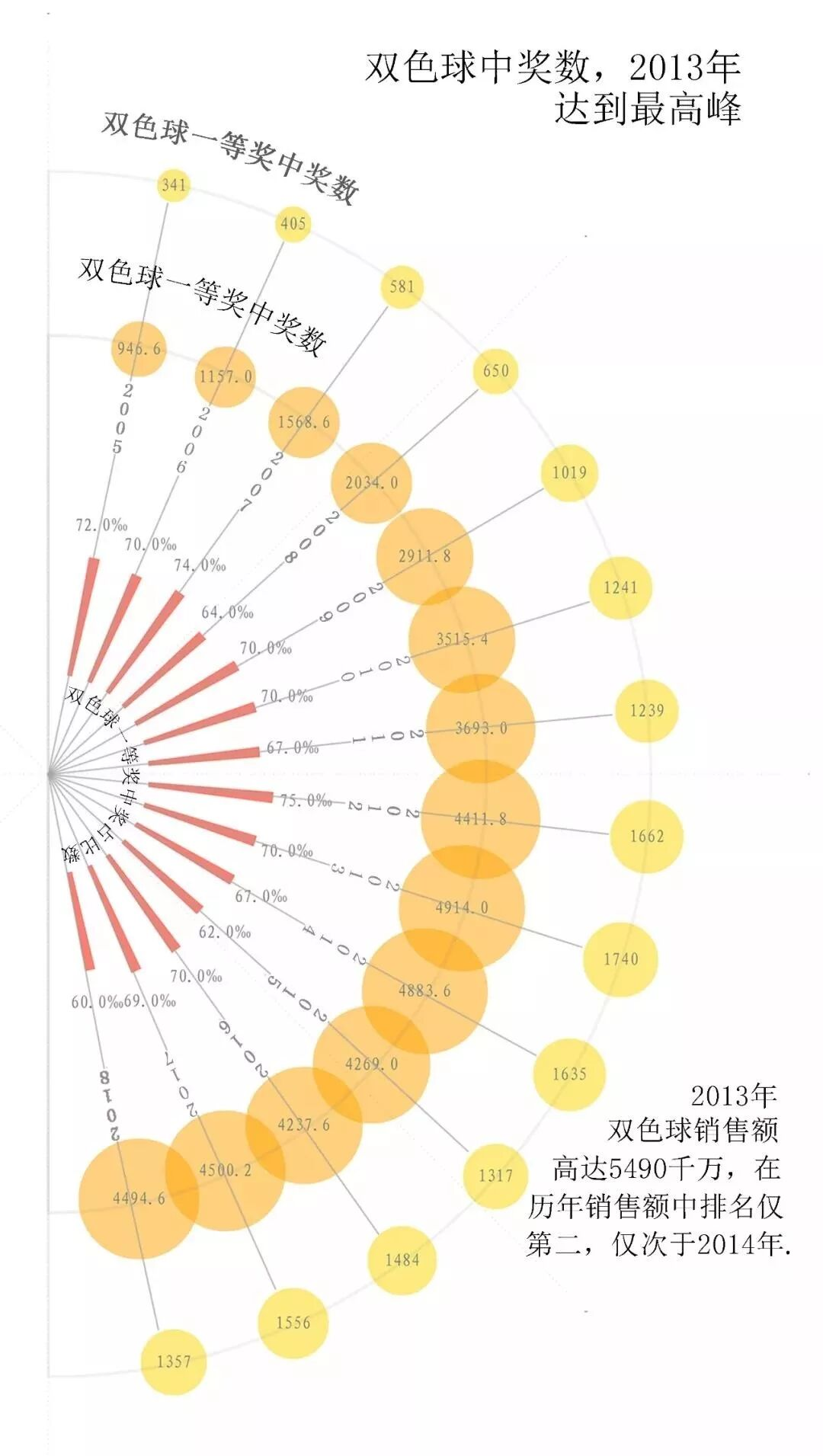▲双色球一等奖中奖占比率一直徘徊在7%左右

04 题外话：为什么穷人更爱买彩票？

▲来源：飞碟说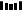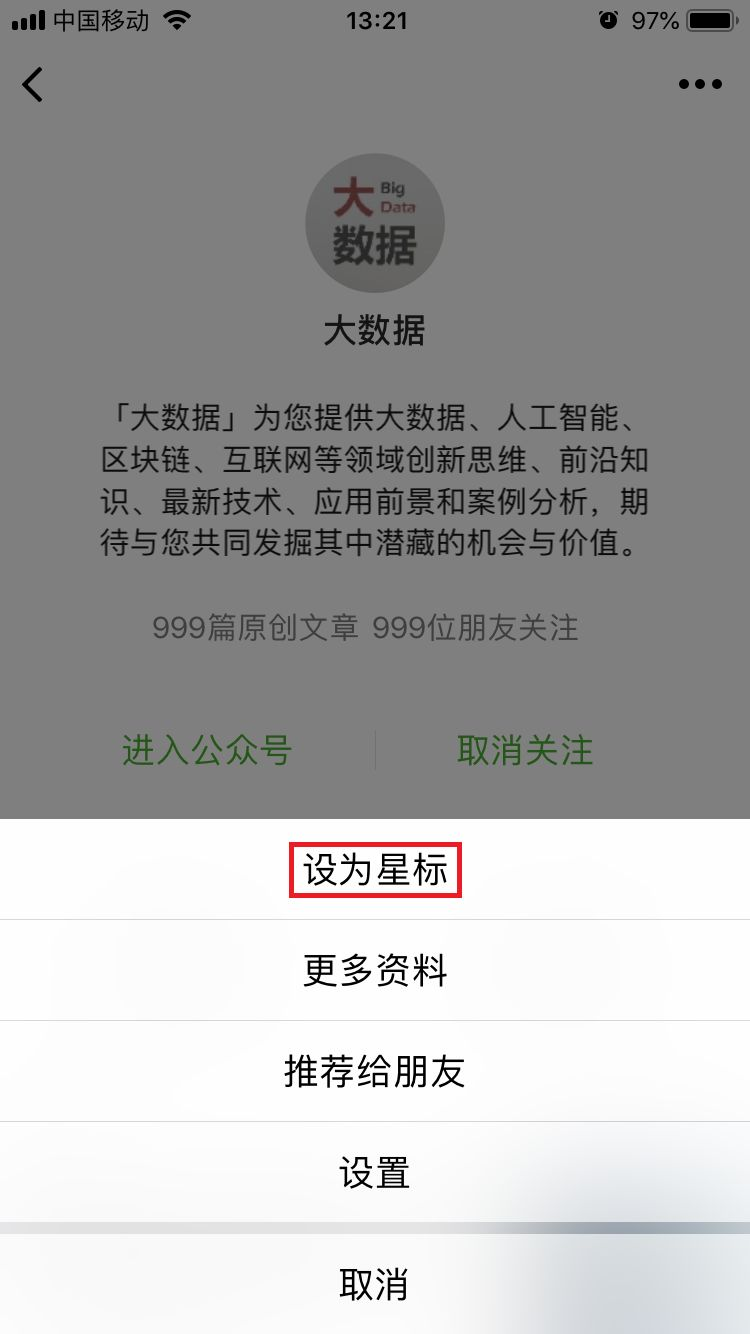PPT | 报告 | 读书 | 书单 | 干货

AI | 1024 | 段子 | 区块链 | 数学

• 什么样的数据才有价值？应该怎样收集和处理？终于有人讲明白了

• 被两会代表频繁“点名”，2019的第一波风口要来了？

• 女神节，来聊聊这几位神一般的“程序媛”

• 马化腾提议加强基础科学研究，中国“芯痛”能解决吗？

Q: 你买彩票中过奖吗？https://www.wxwenku.com/d/110021255